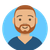# App Development

Announcements
Qlik Insider: SaaS capabilities for Data Integration and Analytics, May 25th! Register Now
cancel
Showing results for
Did you mean:Partner

## Dimension with a conditional sum

Hi to everyone!

I have an issue. I need to create a dimension MONTH that is dependent on two conditions. I want consider the dimension MONTH only if the Measure it is  equal 'Volumes' and its sum on that dimension is = 11.

I tried to write the following expression:

if (Sum({\$<Measure={'Volumes'}>}VALUE)=11, MONTH)

The expression seems to be right, but then the dimension is invalid.

I tried also :

if ([Measure]='Volumes' and VALUE=11, MESE_FISCALE)

that one seems to work in the dimension, but I want the aggregate sum of the VALUE not only the single value.

Thanks to everyone!

Labels (3)

• ### Sum If expression

1 Solution

Accepted SolutionsMaster

the expression
if (Sum({\$<Measure={'Volumes'}>}VALUE)=11, MONTH)
is not allowed in dimension, encapsulate your SUM with AGGR depending on your other dimensions you need to decide what dimensions go inside the AGGR.  try aggr(Sum({\$<Measure={'Volumes'}>}VALUE),MONTH)
also, validate first if the expression will return a value add Sum({\$<Measure={'Volumes'}>}VALUE) as an expression first.

4 RepliesMaster

the expression
if (Sum({\$<Measure={'Volumes'}>}VALUE)=11, MONTH)
is not allowed in dimension, encapsulate your SUM with AGGR depending on your other dimensions you need to decide what dimensions go inside the AGGR.  try aggr(Sum({\$<Measure={'Volumes'}>}VALUE),MONTH)
also, validate first if the expression will return a value add Sum({\$<Measure={'Volumes'}>}VALUE) as an expression first.Luminary Alumni

As @edwin has said, you can't use that kind of expression in a calculated dimension. 99 times out of a 100 when I see this sort of requirement I find it can be solved by having the correct set analysis in your measure expression. Is VALUE a static single value or are you truly trying to sum it? Can you share what measure you are trying to calculate?Partner
Author

Thanks, with your suggestion I resolved my problem!Master

np